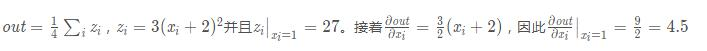欢迎访问优讯网！
 您当前的位置：首页 > 爱编程

variables（变量的序列） - 被求微分的叶子节点，即 ys 。

create_graph（bool，可选） - 如果为True，则将构造派生图，允许计算更高阶的派生产品。默认为False。

 12345678 `import` `torch``from` `torch.autograd ``import` `Variable``x ``=` `Variable(torch.ones(``2``, ``2``), requires_grad``=``True``)``y ``=` `x ``+` `2``z ``=` `y ``*` `y ``*` `3``out ``=` `z.mean()``out.backward()``#这里是默认情况，相当于out.backward(torch.Tensor([1.0]))``print``(x.grad)`

 1234 `Variable containing:`` ``4.5000` `4.5000`` ``4.5000` `4.5000``[torch.FloatTensor of size ``2x2``]`12345678910 `x ``=` `torch.randn(``3``)``x ``=` `Variable(x, requires_grad``=``True``)` `y ``=` `x ``*` `2``while` `y.data.norm() < ``1000``:``  ``y ``=` `y ``*` `2` `gradients ``=` `torch.FloatTensor([``0.1``, ``1.0``, ``0.0001``])``y.backward(gradients)``print``(x.grad)`

 12345 `Variable containing:`` ``204.8000`` ``2048.0000``  ``0.2048``[torch.FloatTensor of size ``3``]`返回首页
 原文链接：https://www.jb51.net/article/177998.htm
 推荐资讯
 相关文章
 无相关信息
 栏目更新
 栏目热门